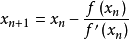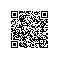# rust练习之高阶过程

rust中参数的函数类型是以trait来实现的，这样的trait有三个，分别是FnOnce,FnMut,Fn

• FnOnce
: 可以调用一次，会转移执行环境中的值
• FnMut
: 可变引用可以调用多次，可以改变执行环境中的值. 继承自FnOnce(所有实现了FnMut的类型也实现了FnOnce).
• Fn
: 共享引用，可以调用多次.既不可以转移，也不可以改变执行环境的值. 继承自FnMut，同样也继承自FnOnce

pub trait Fn<Args> : FnMut<Args> {
extern "rust-call" fn call(&self, args: Args) -> Self::Output;
}
pub trait FnMut<Args> : FnOnce<Args> {
extern "rust-call" fn call_mut(&mut self, args: Args) -> Self::Output;
}
pub trait FnOnce<Args> {
type Output; extern "rust-call" fn call_once(self, args: Args) -> Self::Output;
}//导函数
const DX: f64 = 0.000001;//微分函数的分母，尽可能的小，但由于浮点数的精度限制，取得太小，反而误差会扩大
fn deriv<'a, F>(f: &'a F) -> Box<Fn(f64) -> f64 + 'a>
where F: Fn(f64) -> f64
{
Box::new(move |x| (f(x + DX) - f(x - DX)) / (2.0 * DX))
}


//牛顿迭代法近似
fn newton<'a, F>(f: &'a F) -> Box<Fn(f64) -> f64 + 'a>
where F: Fn(f64) -> f64
{
Box::new(move |x| x - (f(x) / deriv(f)(x)))

}


//函数不动点逼近
fn fixed_point<'a, F, T>(fnt: &'a F, trans: T, first_guess: f64) -> f64
where F: Fn(f64) -> f64,
T: Fn(&'a F) -> Box<Fn(f64) -> f64 + 'a>
{
let tolerance = 0.0000001;
let close_enough = |a: f64, b: f64| (a - b).abs() > tolerance;
let f = trans(fnt);
let mut guess = first_guess;
let mut next: f64 = f(guess);
while close_enough(guess, next) {
// println!("guess next:{}", next);
guess = next;
next = f(next);
}
next
}


fn sqrt(x: f64) -> f64 {

let func = |y: f64| y.powi(2) - x;
fixed_point(&func, newton, 1.0)
}


fn main() {
let x = 2.0;
let func = |y: f64| y.powi(2) - x;
let dx = deriv(&func);
println!(" derivative y^2-x:{}", dx(1.0));
let y = newton;
let nt = y(&func);
println!("newton method:{}", nt(1.4142));
let val = fixed_point(&func, newton, 1.0);
println!("fixed point,sqrt:{}", val);
let x = 3.0;
println!("sqrt(x):{}={}", x, sqrt(x));
}


(define (deriv g)
(lambda (x)
(/ (- (g (+ x dx)) (g x))
dx)))

(define (newton-transform g)
(lambda (x)
(- x (/ (g x) ((deriv g) x)))))

(define tolerance 0.00001)
(define (fixed-point f first-guess)
(define (close-enough? v1 v2)
(< (abs (- v1 v2)) tolerance))
(define (try guess)
(let ((next (f guess)))
(if (close-enough? guess next)
next
(try next))))
(try first-guess))

(define (fixed-point-of-transform g transform guess)
(fixed-point (transform g) guess))

(define (sqrt x)
(fixed-point-of-transform (lambda (y) (- (square y) x))
newton-transform
1.0))使用钉钉扫一扫加入圈子
+ 订阅